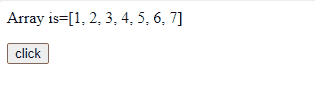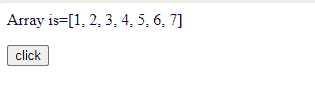# How to shuffle an array using JavaScript ?

Shuffling an array or a list means that we randomly re-arranging the content of that structure. To shuffle an array we will use the following algorithms:

Approach: In this approach, we are using the array elements in reverse order and then swapping each element with a random one.

```function shuffleArray(array) {
for (var i = array.length - 1; i > 0; i--) {

// Generate random number
var j = Math.floor(Math.random() * (i + 1));

var temp = array[i];
array[i] = array[j];
array[j] = temp;
}

return array;
}```

Example: In this example, we are using the above approach.

## html

 `<``p``>Array is=[1, 2, 3, 4, 5, 6, 7]` `<``button` `onclick``=``"show()"``>` `    ``click` ``   `<``script``>` `    ``// Function to shuffle the array content` `    ``function shuffleArray(array) {` `        ``for (var i = array.length - 1; i > 0; i--) {`   `            ``// Generate random number ` `            ``var j = Math.floor(Math.random() * (i + 1));`   `            ``var temp = array[i];` `            ``array[i] = array[j];` `            ``array[j] = temp;` `        ``}`   `        ``return array;` `    ``}`   `    ``// Function to show the result` `    ``function show() {` `        ``var arr = [1, 2, 3, 4, 5, 6, 7]` `        ``var arr1 = shuffleArray(arr)`   `        ``document.write("After shuffling: ", arr1)` `    ``}` ``

Output:How to shuffle an array using JavaScript ?

Approach 2: Passing a function that returns (random value – 0.5 ) as a comparator to sort function, so as to sort elements on a random basis.

```function shuffleArray(array) {
return array.sort( ()=>Math.random()-0.5 );
} ```

Example: This example shows the use of the above-explained approach.

## HTML

 `<``p``>Array is=[1, 2, 3, 4, 5, 6, 7]` `<``button` `onclick``=``"show()"``>` `    ``click` `` `<``script``>` `    ``// Function to shuffle the array content ` `    ``function shuffleArray(array) {` `        ``return array.sort(() => Math.random() - 0.5);` `    ``}`   `    ``// Function to show the result ` `    ``function show() {` `        ``var arr = [1, 2, 3, 4, 5, 6, 7]` `        ``var arr1 = shuffleArray(arr)`   `        ``document.write("After shuffling: ", arr1)` `    ``} ` ``

Output:How to shuffle an array using JavaScript ?

Whether you're preparing for your first job interview or aiming to upskill in this ever-evolving tech landscape, GeeksforGeeks Courses are your key to success. We provide top-quality content at affordable prices, all geared towards accelerating your growth in a time-bound manner. Join the millions we've already empowered, and we're here to do the same for you. Don't miss out - check it out now!

Previous
Next